Courses

# Overview of Periodic Classification of Elements (part -2) Class 10 Notes | EduRev

## Class 10 : Overview of Periodic Classification of Elements (part -2) Class 10 Notes | EduRev

The document Overview of Periodic Classification of Elements (part -2) Class 10 Notes | EduRev is a part of the Class 10 Course Science Class 10.
All you need of Class 10 at this link: Class 10

Position of Elements in the Modern Periodic Table

Modem Periodic Table has 18 vertical columns named as ‘groups’ and 7 horizontal rows known as ‘periods’. Elements with same number of valence electrons are placed in the same group. In other words, a group in a periodic table signifies an identical outer shell electronic configuration.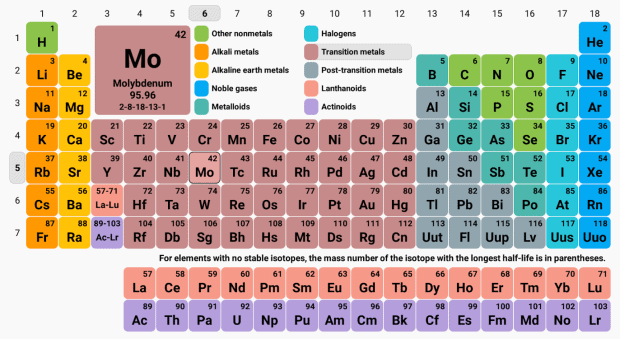Activity: Explain the similarity of group 1 elements.
Consider the elements lithium, sodium and potassium of group 1.
Their electronic configuration may be written as 6: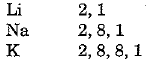Thus, the outermost or valence shell in all three cases contains 1 electron. These elements have been placed in the same group because of this similarity.
Consider the elements Li, Be, B, C, N, O, F and Ne which are present in the second period. The distribution of electrons in these elements can be written as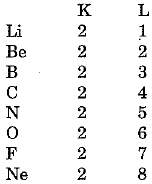We find that the number of electrons in the outermost (valence) shell in the second period elements is not the same. But all these elements contain the same number of shells Le., two. Also we observe that the valence electrons increase successively by one unit. In other words, elements with same number of occupied shells are placed in the same period. Na, Mg, Al, Si, P, S, Cl and Ar belong to the third period because the electrons in these atoms are filled in the three shells, K, L and M.
Maximum number of electrons that can be filled in a shell is given by the 2n2 where n is shell number.
For K-shell n = 1 or 2n2 = 2
Hence, first period has 2 elements.
For L-shell n = 2 or 2n2 = 8
Hence, second period has 8 elements.
For M-shell n = 3 or 2n2, - 1
But the outermost shell can have 8 electrons.
Therefore, the third period can have 8 elements.
The position of an element in the periodic table tells us about its chemical reactivity. Valence electrons determine the kind and number of bonds formed by the element. That is why, Mendeleev made it a basis for classifying elements.

Trends in the Periodic Table

Valency

Valency is given by the number of electrons present in the valence shell i.e., outermost shell.
Activity: To determine the valency of an element.
Let us calculate the valency of Mg with atomic number 12 and valency of sulphur with atomic number 16.
Mg  2, 8, 2
Thus, Mg has a valency of 2.
S  2, 8, 6
Sulphur can form upto 6 bonds.
We observe that valency increases on going from left to right in a period and remains constant in moving from top to bottom in a group.

Atomic size

Atomic size (or atomic radius) may be visualised as the distance between the centre of the atom and the outermost shell of the isolated atom. Atomic radius of hydrogen is 37 pm (1 pm - 10-12 m).
Activity: Study the variation in atomic radii of elements in a period.
The atomic radii of the elements of second period are given below :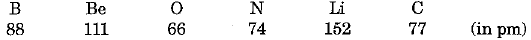(i) They can be arranged in decreasing order of atomic radius as under :
Li > Be > B > C > N > O
(ii) We observe that the above order is the order of arrangement of elements in the second period in the periodic table.
(iii) Lithium has the largest and oxygen has the smallest atom.
Atomic radius decreases as we go from left to right in a period. We can ascribe it to increase in nuclear charge which tends to pull the electron closer to the nucleus so that the size of the atom is reduced.
Activity: Study the variation in atomic size of elements in a group.
The atomic radii of the elements of first group are given below :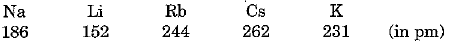(i) The elements can be arranged in increasing order of their atomic radii as under :
Li < Na < K < Rb < Cs
(ii) Lithium has the smallest atoms and caesium has the largest atoms.
(iii) Atomic size increases as we move down the group. This is because new shells are being added as we move down and this increases the distance between the nucleus and outermost electrons. There is an increase in the radius in spite of increase in nuclear charge.

Metallic and Non-metallic Properties

Activity: Classify the elements of third period into metals and non-metals.
The elements of the third period are:
Na Mg Al Si P S Cl Ar
(i) We find that Na, Mg and A1 have tendency to lose electrons, therefore, they behave as metal. Elements like P, S and Cl have a tendency to gain electrons. Therefore, they behave as non-metals.
(ii) Metals occupy the left hand side of the periodic table
(iii) Non-metals occupy right hand side of the periodic table.
In the middle we have silicon which is classified as a semi-metal or metalloid because it exhibits some properties of both metals and non-metals.
Activity: Study the tendency of elements to lose electrons in a group.
(i) The effective nuclear charge acting on valence electrons decreases while moving down the group because the outermost electrons are farther away from the nucleus. Therefore, tendency to lose electrons increases down the group.
(ii) The effective nuclear charge acting on the valence shell electrons increases across a period, because of increased nuclear charge and decreasing size of the atom. Therefore, the tendency to lose electrons decreases in going from left to right in a period. Hence, metallic character decreases across the period but increases down the group.

Offer running on EduRev: Apply code STAYHOME200 to get INR 200 off on our premium plan EduRev Infinity!

## Science Class 10

85 videos|255 docs|129 tests

,

,

,

,

,

,

,

,

,

,

,

,

,

,

,

,

,

,

,

,

,

;